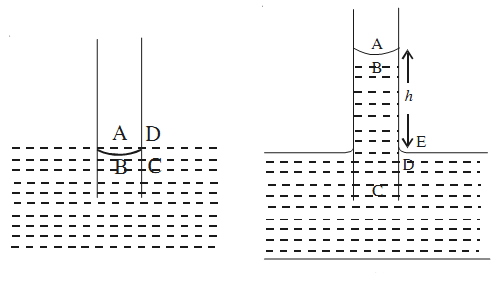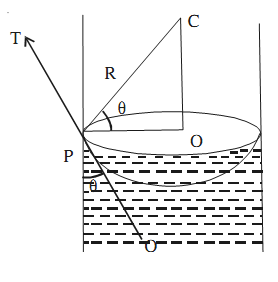# Capillary Action

Blotting paper can be used to absorb extra ink from notebook. The ink rises in the narrow air gaps in the blotting paper. Similarly, if the lower end of a cloth gets wet, water slowly rises upward. Also water given to the fields rises in the innumerable capillaries in the stems of plants and trees and reaches the branches and leaves.

Phenomenon of the elevation or depression of a liquid in an open tube of small cross-section (capillary tube) is basically due to surface tension and is known as capillary action.

The phenomenon of rise or depression of liquids in capillary tubes is known as capillary action or capillarity.

### Rise of Liquid In Capillary Tube

A capillary tube dipped in water. The meniscus inside the tube will be concave. This is essentially because the forces of adhesion between glass and water are greater than cohesive forces.Consider four points A, B, C and D near the liquid-air interface. Pressure just below the meniscus is less than the pressure just above it by 2T/R.

PB = PA – 2T/R

where T is surface tension at liquid-air interface and R is the radius of concave surface.

But pressure at A is equal to the pressure at D and is equal to the atmospheric pressure P. Pressure at D is equal to pressure at C . Therefore, pressure at B is less than pressure at D.

But the pressure at all points at the same level in a liquid must be same. That’s why water begins to flow from the outside region into the tube to make up the deficiency of pressure at point B. Thus, liquid begins to rise in the capillary tube to a certain height h till the pressure of liquid column of height h becomes equal to 2T/R. Thereafter, water stops rising. In this condition

h ρ g = 2 T/R

where ρ is the density of the liquid and g is the acceleration due to gravity. If r be radius of capillary tube and θ be the angle of contact, then

R = r/cosθh = 2T cosθ / r ρ g

If the radius of tube is less (in a very fine bore capillary), liquid rise will be high.